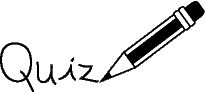Created 06/12/03

#on Number Representation

Instructions: For each question, choose the single best answer. Make your choice by clicking on its button. You can change your answers at any time. When the quiz is graded, the correct answers will appear in the box after each question.

1. Which of the following could represent the number eight?

 A.    8 B.    //// //// C.    iix D.    They all could represent the number.

2. The number thirteen is a prime number, a number that has no divisors other than itself and one. Which of the following represent a prime number?

 A.    13 B.    XIII C.    11012 D.    All of them. The properties of a number do not depend on how it is represented.

3. What are some of the advantages of positional notation?

 A.    Compact, easy to compute with. B.    Uses familiar characters, always uses base ten. C.    Does not need a symbol for zero, easy to learn. D.    Numbers of any size can be represented, more accurate than other methods.

4. What is the value of B0, where B is an integer?

 A.    0 B.    1 C.    2 D.    B

5. What are the "digits" ordinarily used for base five?

 A.    0 1 2 3 4 B.    1 2 3 4 5 C.    0 1 2 3 4 5 D.    a b c d e

6. Here is a number represented in base three. The expression on the right of = is in base ten. What should fill the blanks?

```2013 = 2 × 3--- +  0 × 3--- + 1 × 3---
```
 A.    `2 1 0` B.    `3 2 1` C.    `1 2 3` D.    `0 1 2`

7. Convert the following to base ten: 2013

 A.    5 B.    8 C.    19 D.    98 E.    105

8. Change the representation of `2034` from base four to base ten.

 A.    510 B.    1110 C.    1910 D.    3510 E.    6710

9. What are the "digits" of base two number representations usually called?

 A.    bits B.    bytes C.    quarks D.    dots

10. Change the representation of `102` from base two to base ten.

 A.    0 B.    1 C.    2 D.    3 E.    4 F.    5

11. Change the representation of `1012` from base two to base ten.

 A.    0 B.    1 C.    2 D.    3 E.    4 F.    5

12. Change the representation of `10112` from base two to base ten.

 A.    `310` B.    `810` C.    `1110` D.    `1410` E.    `1910` F.    `3210`

13. Change the representation of `248` from base eight to base ten.

 A.    `710` B.    `1210` C.    `1710` D.    `2010` E.    `2710` F.    `3210`

14. Change the representation of `0101002` from base two to base ten.

 A.    `1710` B.    `2010` C.    `2110` D.    `2610` E.    `2810` F.    `3210`

15. Change the representation of `248` from base eight to base two (hint: look at the previous two questions.)

 A.    `0001102` B.    `0101102` C.    `0101002` D.    `0100102` E.    `0101112` F.    `1101102`

The number you got right:       Percent Correct:       Letter Grade:

If you have returned here from another page, or have re-loaded this page, you will need to click again on each of your choices for the grading program to work correctly. You may want to press the SHIFT KEY while clicking to clear the old answers.